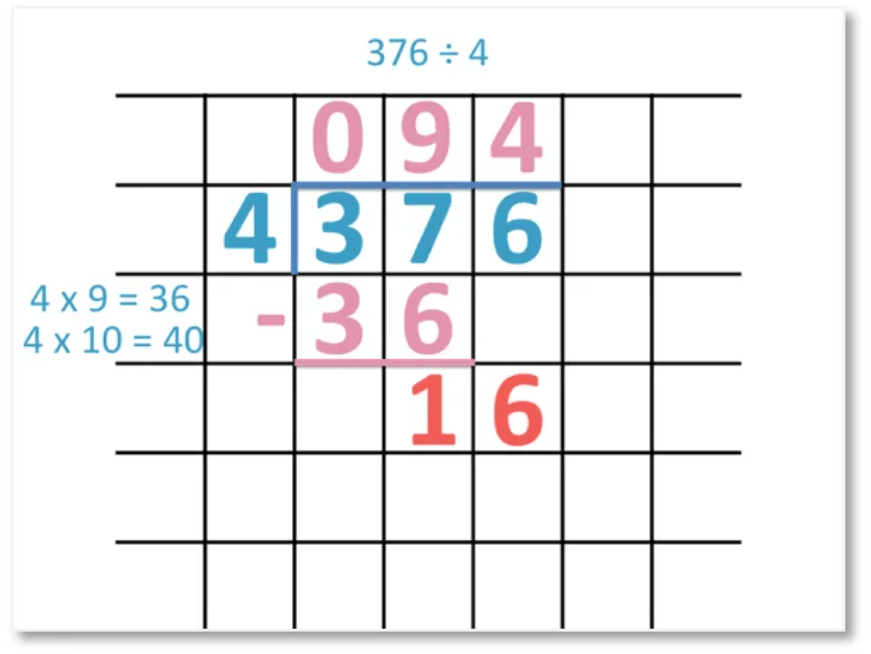# Short Division with Decimal Remainders

Short Division with Decimal Remainders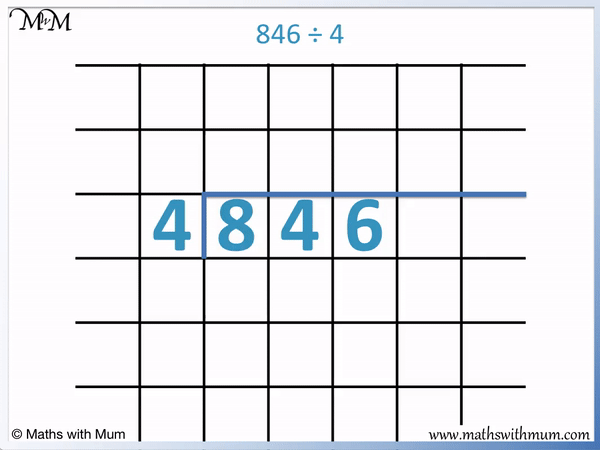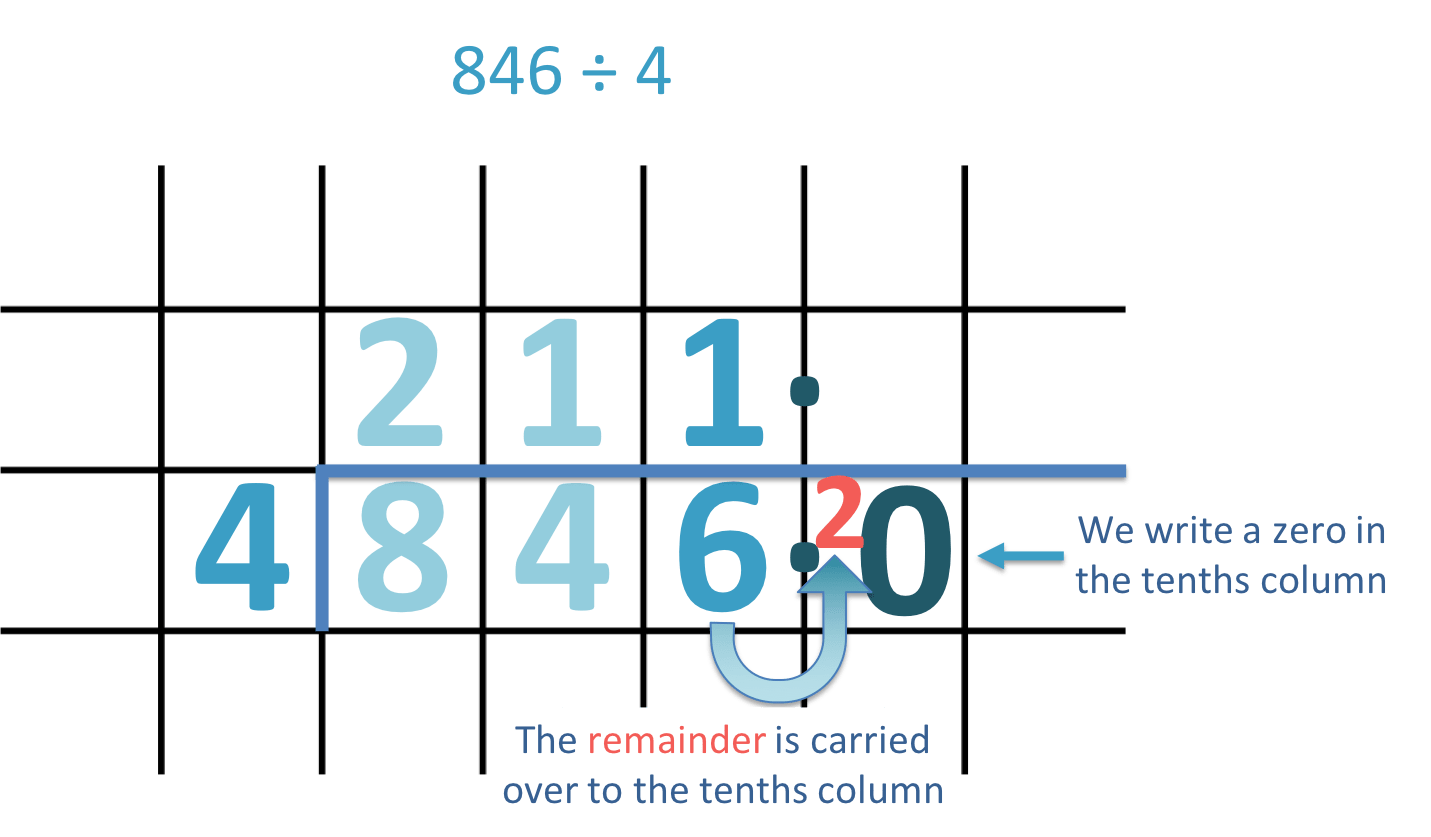• To perform schort division, divide each in the number by the digit written on the left.
• We divide each digit in 846 by 4, working from to left to right.
• 8 ÷ 4 = 2. We write the 2 above the line.
• Next, 4 ÷ 4 = 1 and we write this above the line.
• 6 ÷ 4 = 1 remainder 2. This is because we need 2 more from 4 to make 6.
• This remainder is carried over to the tenths column.
• Since there are no tenths in the original number of 846, we write a zero in the tenths column.
• Now we can carry the remainder of 2 over to make ’20’ tenths, which can then be divided by 4.
• 20 ÷ 4 = 5 and so, we write 5 in the tenths column above the line.
• 846 ÷ 4 = 211.5.
Write a zero after the decimal point and carry the remainder over for division.• 4 ÷ 4 = 1.
• 8 ÷ 4 = 2.
• 7 ÷ 4 = 1 remainder 3.
• Since we have a remainder, we need to write a zero in the first decimal column and carry the remainder of 3 over to make 30 tenths.
• 30 ÷ 4 = 7 remainder 2.
• Since we still have a remainder, we write another zero in the next column along and carry the 2 over to make 20 hundredths
• 20 ÷ 4 = 5. Since there are no more remainders, we have our final answer.
• 487 ÷ 4 = 121.75.# Short Division with Decimal Remainders

## How to do Short Division with Decimal Remainders

To do short division with decimal remainders, follow these steps:

1. Divide each digit individually by looking at the digits from right to left.
2. Write the greatest number of times that the number divides into each digit above the line.
3. Write the remainder in front of the next digit to the right.
4. Look at the next column along and repeat steps 1 to 3.
5. If there is not a digit in the next column along, write the remainder in front of a 0.

Decimal remainders occur when a number does not divide exactly into another. The remainder of the division is written in front of zero digits written after the decimal point.

Here is an example of short division with a decimal remainder. We have 561 ÷ 5.

To do short division, write the number being divided by on the left of the number being divided. Draw a line between the numbers that also goes over the top of the number being divided.

We write the digit of 5 to the left of the number 561.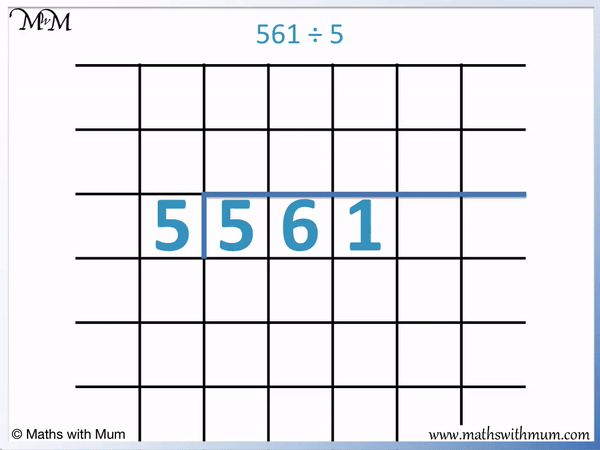The first step is to divide each digit from right to left. Write the answer above the line

5 ÷ 5 = 1 and so, 1 is written above the line.

6 ÷ 5 = 1 remainder 1. Because 5 goes into 6 once, a 1 is written above the line. The remainder is carried over in front of the next digit along. The 1 is written in front of the 1 in 561.

We now divide ’11’ by 5. 11 ÷ 5 = 2 remainder 1. 5 goes into 11 twice and so, we write 2 above the line.

Since there is a remainder, it must be carried across to the next place value column. There is no digit in the decimal place value columns.

If there is no digit in the decimal place value columns, write a zero. The remainder is then written in front of the zero digit.

The remainder of 1 is written in front of the zero in the tenths column to make ’10’.

We then divide 10 by 5. 10 ÷ 5 = 2 and so, we write a 2 above the line.

The decimal point of the answer is written directly between the ones digit and the tenths digit of the answer.

561 ÷ 5 = 112.2

## How to Write Remainders as Decimals

To write a remainder as a decimal, follow these steps:

1. Write a zero in the place value column immediately after the decimal point.
2. Write the remainder from the ones column in front of the zero to make a 2-digit number.
3. Divide this 2-digit number by the number in the question.
4. If there is still a remainder, write another zero in the next column and place the remainder in front of it.
5. Repeat this last step and divide by the number in question until there are no more remainders.

A remainder is the amount left over after a division. It is the difference between the number being divided and the largest multiple of the number being divided by. For example 9 ÷ 4 = 2 remainder 1 because the largest multiple of 4 that goes into 9 is 8 and the difference between 9 and 8 is 1.

Here is an example of writing a remainder as a decimal. We have 846 ÷ 4.

If the number is not exactly divisible by the other number, a remainder can be written.

The first step is to write a zero after the decimal point. We write 846 as 846.0.

The division of each digit is done from right to left.

8 ÷ 4 = 2

4 ÷ 4 = 1

6 ÷ 4 = 1 remainder 2. This is because 4 goes into 6 once with 2 left over. There is a difference of 2 between 6 and 4.

The remainder from the ones column is written in front of the zero in the tenths decimal column.We write the remainder of 2 in front of the 0 in the decimal column to make ’20’.

We now divide 20 by 4. 20 ÷ 4 = 5 and so, 5 is written above the line after the decimal point.

The decimal remainder must be written after the decimal point.846 ÷ 4 = 211.5

Here is another example of writing remainders as decimals.

We have 487 ÷ 4.Divide each digit in the number individually, working from right to left.

4 ÷ 4 = 1 and 8 ÷ 4 = 2. These divisions are exact and there are no remainders.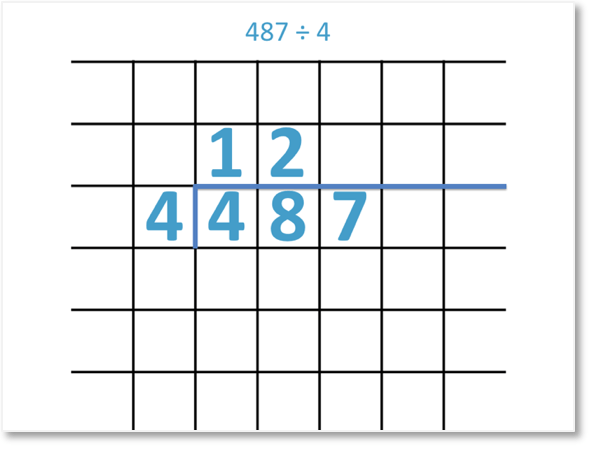Next we divide 7 by 4. 4 goes into 7 once with 3 left over. The remainder is 3 because the difference between 7 and 4 is 3.

If there is a remainder from the ones place value column, a zero must be written in the tenths place value column. The remainder is then written in front of this zero.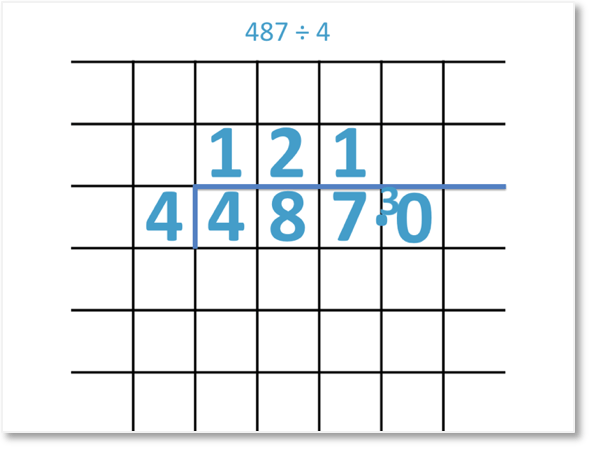The remainder of 3 is written in front of the 0 digit to make 30. We now divide 30 by 4.

30 ÷ 4 = 7 remainder 2. This is because 7 fours are 28 and the difference between 30 and 28 is 2.

The 7 is written above the line and the remainder of 2 must be carried over to the next column. We need to write a zero in first.

A zero must be written in a place value column if a remainder is to be carried over to this column and there is currenly no digit written in the column.The digit of 2 is moved over to the 0 to make 20.

We now divide 20 by 4 to make 5. This is an exact division and so we have arrived at our answer.

487 ÷ 4 = 121.75

Once all digits have been divided and there is no longer a remainder, the short division method has been completed.Now try our lesson on Introduction to Long Division where we learn how to perform even larger division calculations using a method known as long division.